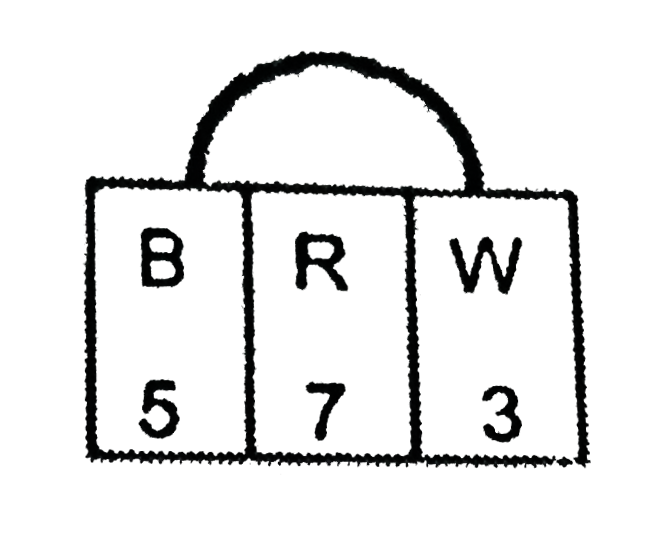# A bag contains 5 black, 7 red and 3 white balls. A ball is drawn from the bag at random. Find the probability that the ball drawn is : (i) red (ii) bl

41 views

closed
A bag contains 5 black, 7 red and 3 white balls. A ball is drawn from the bag at random.
Find the probability that the ball drawn is :
(i) red (ii) black or white (iii) not black.

by (71.5k points)
selected by

Total number of balls = 5 + 7 +3 = 15
(i) Number of red balls = 7
therefore "P(drawing a red ball) "=(7)/(15)
(ii) Number of black or white balls = 5 + 3 = 8
therefore "P(drawing a black or white ball) "=(8)/(15)
(iii) Number of balls which are not black = 15 - 5 = 10
therefore "P(drawing a ball that is not black) "=(10)/(5)=(2)/(3)
Hence, the probability of getting a red ball, a black or white ball and not black ball are (7)/(15),(8)/(15)and (2)/(3)" respectively."